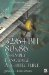# Exercises1. Write a sincos function in assembly language similar to sincosf but using double-precision floating-point.

2. Write a floating-point equation A+B—C+D using RPN. Write it using FPU instructions.

3. Write a factorial program to solve for !15 using FPU instructions and taking advantage of the stack.

4. How much accuracy does the vmp_IsFEqual() function allow with a single-precision definition? Fast precision?

5. Does vmp_IsFEqual() accept a negative value for the third argument? Should there be an assertion? Why or why not?

6. In this chapter, vmp_IsVEqual() uses an ASSERT_PTR4(). What assertion would be used instead to force a 16-byte alignment?

7. Write C functions to support double-precision for:

1. vmp_IsDEqual() scalar double-precision

2. vmp_IsDVEqual() vector double-precision32/64-Bit 80x86 Assembly Language Architecture
ISBN: 1598220020
EAN: 2147483647
Year: 2003
Pages: 191

Similar book on Amazon